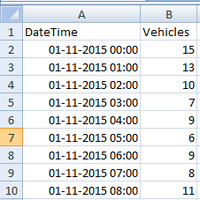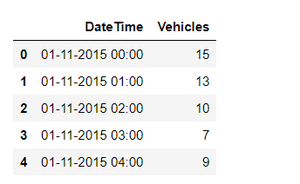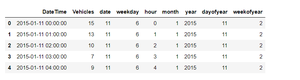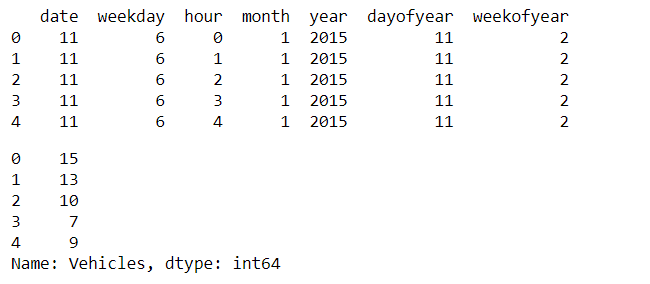# Vehicle Count Prediction From Sensor Data

• Last Updated : 12 Oct, 2021

Sensors which are placed in road junctions collect the data of no of vehicles at different junctions and gives data to the transport manager. Now our task is to predict the total no of vehicles based on sensor data.

Attention reader! Don’t stop learning now. Get hold of all the important Machine Learning Concepts with the Machine Learning Foundation Course at a student-friendly price and become industry ready.

This article explains how to deal with sensor data given with a timestamp and predict the count of the vehicle at a particular time,

Data Set Description:

This dataset contains 2  attributes. They are Datetime and Vehicles. Where Vehicles is the class label.The class label is of numeric type. So the regression technique is well suited for this problem. Regression is used to map the data into a predefined function it is a supervised learning algorithm that is used to predict the value based on historical data. We can perform regression on our data if the data is numeric. Here class label Ie Vehicles attribute is the class label which is numeric so regression should be done.

Random Forest Regressor is an ensemble technique that takes the input and builds trees then takes the mean value of all trees per row/per tuple.

Syntax: RandomForestRegressor(n_estimators=100, *, criterion=’mse’, max_depth=None, min_samples_split=2, min_samples_leaf=1, min_weight_fraction_leaf=0.0, max_features=’auto’, max_leaf_nodes=None,min_impurity_decrease=0.0, min_impurity_split=None, bootstrap=True, oob_score=False, n_jobs=None,random_state=None, verbose=0, warm_start=False, ccp_alpha=0.0, max_samples=None)

Approach:

• Import necessary modules
• Analyze the data
• Convert DateTime attribute to week, days, hours, month, etc (which is in timestamp format.)
• Build the model
• Train the model
• Test the data
• Predict the results

## Python3

 `# importing the pandas module for``# data frame``import` `pandas as pd`  `# load the data set into train variable.``train ``=` `pd.read_csv(``'vehicles.csv'``)` `# display top 5 values of data set``train.head()`

Output:Step 2: Define the functions for getting month, day, hours from the Timestamp (DateTime) and load it into different columns.

## Python3

 `# function to get all data from time stamp` `# get date``def` `get_dom(dt):``    ``return` `dt.day` `# get week day``def` `get_weekday(dt):``    ``return` `dt.weekday()` `# get hour``def` `get_hour(dt):``    ``return` `dt.hour` `# get year``def` `get_year(dt):``    ``return` `dt.year` `# get month``def` `get_month(dt):``    ``return` `dt.month` `# get year day``def` `get_dayofyear(dt):``    ``return` `dt.dayofyear` `# get year week``def` `get_weekofyear(dt):``    ``return` `dt.weekofyear`  `train[``'DateTime'``] ``=` `train[``'DateTime'``].``map``(pd.to_datetime)``train[``'date'``] ``=` `train[``'DateTime'``].``map``(get_dom)``train[``'weekday'``] ``=` `train[``'DateTime'``].``map``(get_weekday)``train[``'hour'``] ``=` `train[``'DateTime'``].``map``(get_hour)``train[``'month'``] ``=` `train[``'DateTime'``].``map``(get_month)``train[``'year'``] ``=` `train[``'DateTime'``].``map``(get_year)``train[``'dayofyear'``] ``=` `train[``'DateTime'``].``map``(get_dayofyear)``train[``'weekofyear'``] ``=` `train[``'DateTime'``].``map``(get_weekofyear)` `# display``train.head()`

Output:Step 3: Separate the class label and store into the target variable

## Python3

 `# there is no use of DateTime module``# so remove it``train ``=` `train.drop([``'DateTime'``], axis``=``1``)` `# separating class label for training the data``train1 ``=` `train.drop([``'Vehicles'``], axis``=``1``)` `# class label is stored in target``target ``=` `train[``'Vehicles'``]` `print``(train1.head())``target.head()`

Output:Step 4: Create and train the data using Machine Learning algorithms and predict the results after testing.

## Python3

 `#importing Random forest``from` `sklearn.ensemble ``import` `RandomForestRegressor` `#defining the RandomForestRegressor``m1``=``RandomForestRegressor()` `m1.fit(train1,target)``#testing``m1.predict([[``11``,``6``,``0``,``1``,``2015``,``11``,``2``]])`

Output:

`array([9.88021429])`

My Personal Notes arrow_drop_up